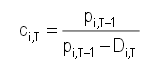# Price correction factors for ALR and OIL shares in BET-XT-TR index starting with May 14th

5/11/2018

Bucharest Stock Exchange (BVB) announces that, starting with 14th of May 2018,

• the price correction factor ci,T for ALRO S.A. (ALR) which will be considered in calculating the BET-XT-TR index is 1.144862.
• the price correction factor ci,T for OIL TERMINAL S.A. (OIL) which will be considered in calculating the BET-XT-TR index is 1.050869.

The values of ci,T for ALR and OIL were determined according to the 4.2.4 article of BVB index management rules:

“4.2.4 Distribution of cash dividends

If an index constituent decides to distribute cash dividends to its shareholders, regardless of their source, the price correction factor ci,T is determined as follows:where

Pi,T-1  : closing price of symbol i at time T-1;

Di,T  : dividend payable for symbol i, for which time T is the ex-date”